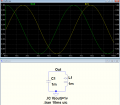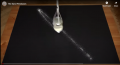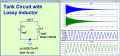# Resonance frequency

#### james7701

Joined Jan 5, 2016
46
Hi... this may be a little silly, I understand that when inductive reactance and capacitive reactance is equal, they cancel out. But, what exactly happens at the resonate frequency?

#### Papabravo

Joined Feb 24, 2006
19,558
There is an echange of energy between the magnetic field in the inductor and the electric field in the capacitor.

#### james7701

Joined Jan 5, 2016
46
An exchange of energy? So, does this exchange form a sine wave, like in an oscillator?

#### WBahn

Joined Mar 31, 2012
27,854
An exchange of energy? So, does this exchange form a sine wave, like in an oscillator?
Not sure what you mean by "like an oscillator". Not all oscillators form sine waves -- that's why we have things that are specifically known as "sine wave oscillators".

To correct one thing in your original post, you said when the inductive reactance and the capacitive reactance are equal. They can't be equal because inductive reactance is positive while capacitive reactance is negative. Neglecting resistance and its effects (which is not always possible), it's when the inductive and capacitive reactances are equal and opposite. For an inductor and capacitor in series at resonance, this means that the total reactance goes to zero when they are added. If they are in parallel, it means that their total reactance becomes infinite when their reciprocals are added.

Let's focus on a series-connected inductor and capacitor with a sinusoidal current flowing in them (since they are in series, whatever current is flowing in the inductor at a given moment is the same current that is flowing in the capacitor (assuming that the frequency is low enough that we don't have to consider transmission line effects, which is likely a good assumption until we get into the hundreds of megahertz).

As you likely know, the energy stored in the magnetic field of an inductor is proportional to the square of the current flowing through it, while the energy stored in a capacitor is proportional to the square of the voltage appearing across it. If the current is sinusoidal, then the energy in each device is building to a peak and dropping to zero twice per cycle. But the voltage across the capacitor is ninety degrees out of phase with the current in the inductor. As a result, the peak energy stored in the inductor occurs when there is minimum energy stored in the capacitor and vice-versa. This is the case at any frequency, but the peak energy stored in both is not the same, so the external circuit must periodically absorb the excess energy and then supply it back (this is the reactive power that must be supplied). But at resonance, there is just enough energy between the two that it shuttles back and forth between them without needing the external circuit to provide any makeup or absorb any excess.

#### crutschow

Joined Mar 14, 2008
31,097
Below is the LTspice simulation of the resonant sinewave oscillation of an ideal (no resistance) inductor in parallel with a capacitor:
Note that when the capacitor voltage (green trace) reaches the maximum, the inductor current (yellow trace) is zero, and when the inductor current reaches the maximum, the capacitor voltage is zero, showing the transfer of energy back and forth between the capacitor (½CV²) and the inductor (½LI²).
If you calculate the peak energy of each (1V for capacitor, and 1A for inductor) you will see they are equal).Last edited:
•k1ng 1337

#### eetech00

Joined Jun 8, 2013
3,418
To the TS (in case its not understood):

The initial charge voltage in the simulation shown in post #5 comes from the statement ".IC V(out)=1v".
This statement initially applies 1V to both L1 and C1 (via net "out") at simulation startup.

•crutschow

#### ronsimpson

Joined Oct 7, 2019
2,515
I can't find the video I want to show but this might do. Here is a bucket of sand with a hole in it. When the bucked is hanging down it has no energy or speed. It is dropping sand in the center of the black paper. If you moved the paper to one side slowly you have an oscilloscope. sand art
By hand pull the bucket to one side and you added energy to the bucket. Let go and the bucket heads to center trading energy for speed. As the bucket passes zero/center it starts slowing down while it collects negative energy. When the bucket stops on the other side it is full of - energy and has no speed. (maybe I should say pull to the left or pull to the right energy)The capacitor and inductor act much like the sand bucket. A capacitor can be charged up with energy/voltage. The energy runs back and forth from C to L and back again in a sine wave.
In a capacitor voltage^2 is energy, in an inductor current^2 is energy.

#### Ramussons

Joined May 3, 2013
1,321
An exchange of energy? So, does this exchange form a sine wave, like in an oscillator?
Yes, the energy exchange takes place in a Sinosoidal fashion. The plot he Electric field / Magnetic field Vs Time will be Sinosoidal. aka an oscillator.

#### Papabravo

Joined Feb 24, 2006
19,558
The following slight modification of the circuit presented by @crutschow adds a small bit of series resistance to the inductor and we have the classical RLC circuit characterized by sinusoidal waveforms with a decaying exponential envelope. Without an external source of power, the tiny resistance in a real component will eventually dissipate all of the energy provided by the initial condition.